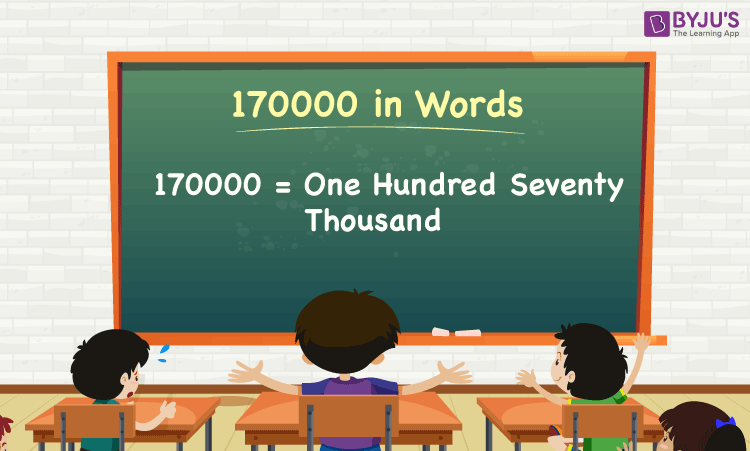# 170000 in Words

170000 in words is One Hundred Seventy Thousand. In Mathematics, the number name of any number can be written with the help of a place value chart. For instance, if you purchased a bike that costs Rs. 170000, you can write it as, “I have purchased a bike that costs Rs. One Hundred Seventy Thousand”.

 170000 in Words: One Hundred Seventy Thousand

Thus, the place value chart is useful for writing the number 170000 in words. In this article, let us discuss how to write the spelling of 170000, and look at the solved examples in detail.

## How to Write 170000 in Words?To write 170000 in words, determine the place value of each digit of the given number 170000.

I.e.,

1’s place of 170000 = 0

10’s place of 170000 = 0.

100’s place of 170000 = 0.

1000’s place of 170000 = 170.

The place value chart for the number 170000 is as follows:

 Thousands Hundreds Tens Ones 170 0 0 0

Hence, 170000 in words is One Hundred Seventy Thousand.

### Examples

Example 1:

Simplify the expression 150000 + 20000, and express the value in words.

Solution:

Given expression: 150000 + 20000

⇒ 150000 + 20000 = 170000

So, the value of 150000 + 20000 is 170000.

Therefore, 170000 in words is One Hundred Seventy Thousand.

Example 2:

Find the sum of two hundred ten thousand and one hundred seventy thousand. Write the sum in words.

Solution:

Two hundred ten thousand = 210000

One hundred seventy thousand = 170000

Thus, two hundred ten thousand plus one hundred seventy thousand = 210000 + 170000 = 380000

Therefore, the sum of two hundred ten thousand and one hundred seventy thousand is three hundred eighty thousand in words.

Register with BYJU’S – The Learning App, and download the app to learn all Maths concepts quickly.

## Frequently Asked Questions on 170000 in Words

Q1

### Write 170000 in words.

170000 in words is one hundred seventy thousand.

Q2

### Express the value 175000 – 5000 in words.

Simplifying 175000 – 5000, we get 170000. Hence, 170000 in words is one hundred seventy thousand.

Q3

### Write one hundred seventy thousand in numbers?

One hundred seventy thousand in numbers is 170000.

Q4

### Is the number 170000 a composite number?

Yes, 170000 is a composite number.

Q5

### Is 170000 a perfect square number?

No, 170000 is not a perfect square number.